### IMO Shortlist 1996 problem G4

Kvaliteta:
Avg: 0,0
Težina:
Avg: 7,0
Let$ABC$ be an equilateral triangle and let$P$ be a point in its interior. Let the lines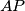$AP$,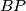$BP$,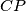$CP$ meet the sides$BC$,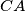$CA$,$AB$ at the points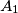$A_1$,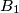$B_1$,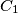$C_1$, respectively. Prove that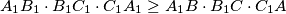$A_1B_1 \cdot B_1C_1 \cdot C_1A_1 \ge A_1B \cdot B_1C \cdot C_1A$.
Izvor: Međunarodna matematička olimpijada, shortlist 1996